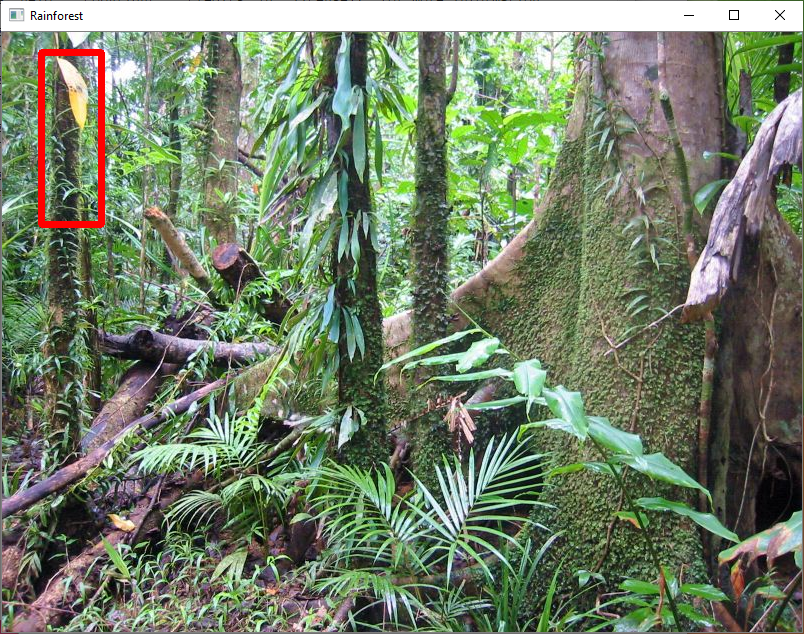﻿# How to Match an Image That Is Embedded in Another Image in Python using OpenCVIn this article, we show how to match an image that is embedded in another image in Python using the OpenCV module.

OpenCV has functions that can perform targeted searches throughout a larger image for an image embedded in it, assuming we feed it the target image to search for.

This is called template matching.

OpenCV has a function, cv2.matchTemplate(), which allows us to look for an image embedded in another (larger) image, and we can see if we can find this.

This can have many useful applications such as discovering if an object is located within a very cumbersome image.

So, in this example, let's say, we have the following image shown below.We now have a portion of this image as a standalone image shown below. We took out this portion out of the original image. This represents the yellow leaf.So we have taken this portion out of the original Rainforest image. This image contains a yellowing leaf.

Using OpenCV, we locate the target image within the larger image we are searching for it in and draw a rectangle around the match.

This gives us the following image shown below.So let's now go to the code to see how this is done. The full code is shown below.

Let's now go over this code.

We import OpenCV module.

We then create a variable, image, which contains the image we want to search. In this case, it is 'Rainforest.png'.

We show this image.

We then create a grayscale version of this image. This simplifies the image.

We then create another variable, template, which represents the subset image that we want to search for within the larger image, image ('Rainforest.png'). This image is named 'Yellowing-leaf.png'.

We then have another variable, result, which stores basically whether there is a match found.

We then create a tuple of values that allows us to get the location of the match, assuming there is a match. The variable, max_loc, represents the

Next, we have another tuple, height and width, that contain the height and width of the template image, or the target image we are searching for within the larger image. We have these values because we are going to highlight the image once found.

We then have a variable, opposite_corner, which represents the bottom right of the rectangle we will create. If we take max_loc and add the width and max_loc and add the height, we have obtained the bottom right corner.

max_loc represents the top left corner.

We then use the cv2.rectangle() function to draw a rectangle around the match. We do this in a red color with a line thickness of 5.

We then show the image.

And this is how we can match an image embedded in another image in Python using OpenCV.

Related Resources